# NCERT Exemplar Solutions for Class 9 Maths Chapter 6 - Lines And Angles

## NCERT Exemplar Solutions Class 9 Maths Chapter 6 – Free PDF Download

NCERT Exemplar Class 9 Mathematics Chapter 6 Lines and Angles, is provided in PDF format for students to prepare for exams. Our experts have designed exemplar problems in accordance with CBSE syllabus (2021-2022) for 9th standard, which covers the following topics of chapter Lines and Angles given below;

• Basic terms like line-segment, collinear points, non-collinear points, right angle, straight angle, acute angle, reflex angle, complementary angles, etc.
• Lines which are intersecting and non-intersecting
• Linear pair of angles such as adjacent angles, vertically opposite angles, etc.
• Transversal intersecting Parallel lines forming angles such as exterior angles, consecutive interior angle.
• Lines parallel to the same line
• Angle Sum Property of a Triangle

This chapter is divided into two parts in the first part the students will learn about lines and in the second part, the students will learn about different angles. Learning the concepts of lines and angles is very much important to understand the concepts of geometry in Class 9 as well as in Class 10. To make understand these concepts, free  NCERT Exemplar Class 9 Maths are provided here.

## Download PDF of NCERT Exemplar Solutions for Class 9 Maths Chapter 6 Lines and Angles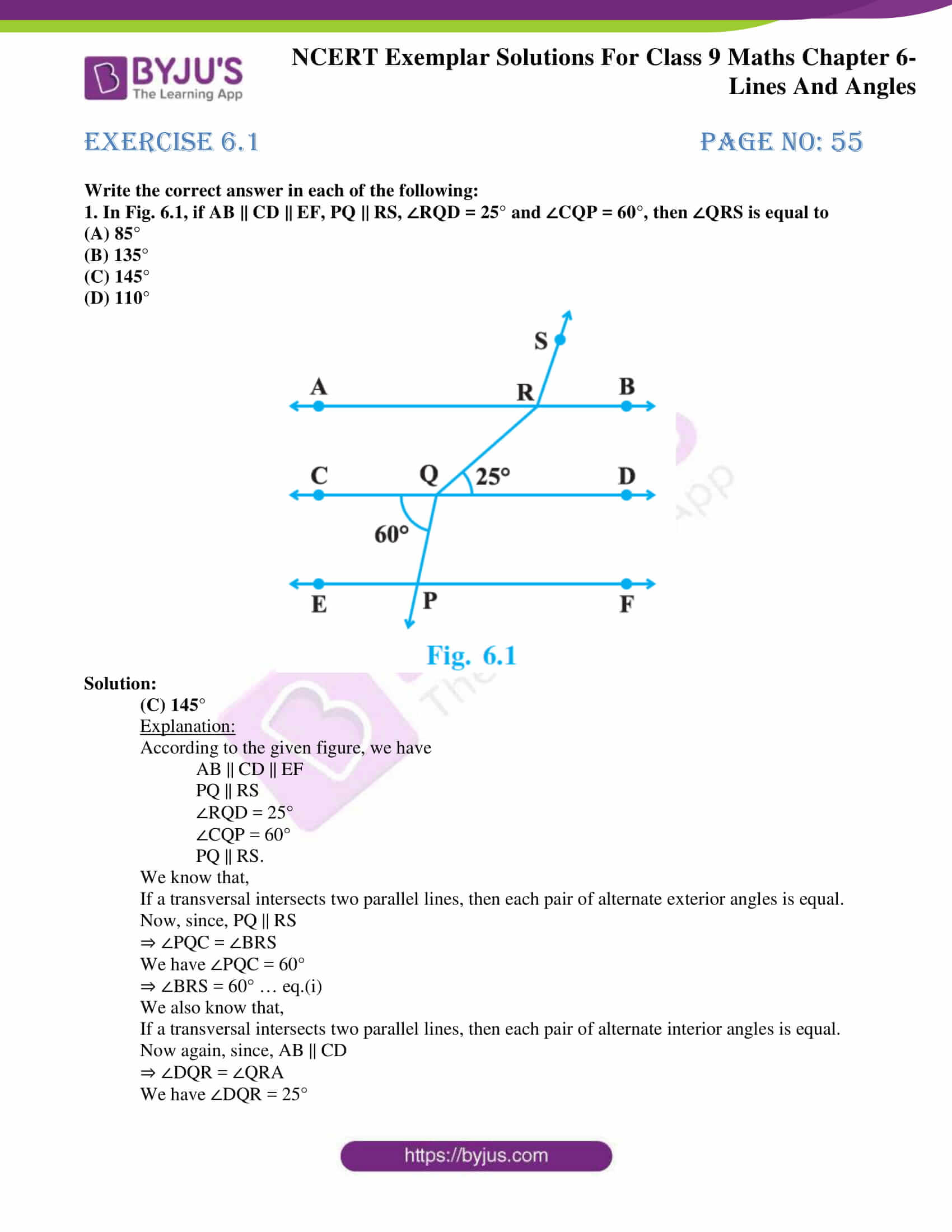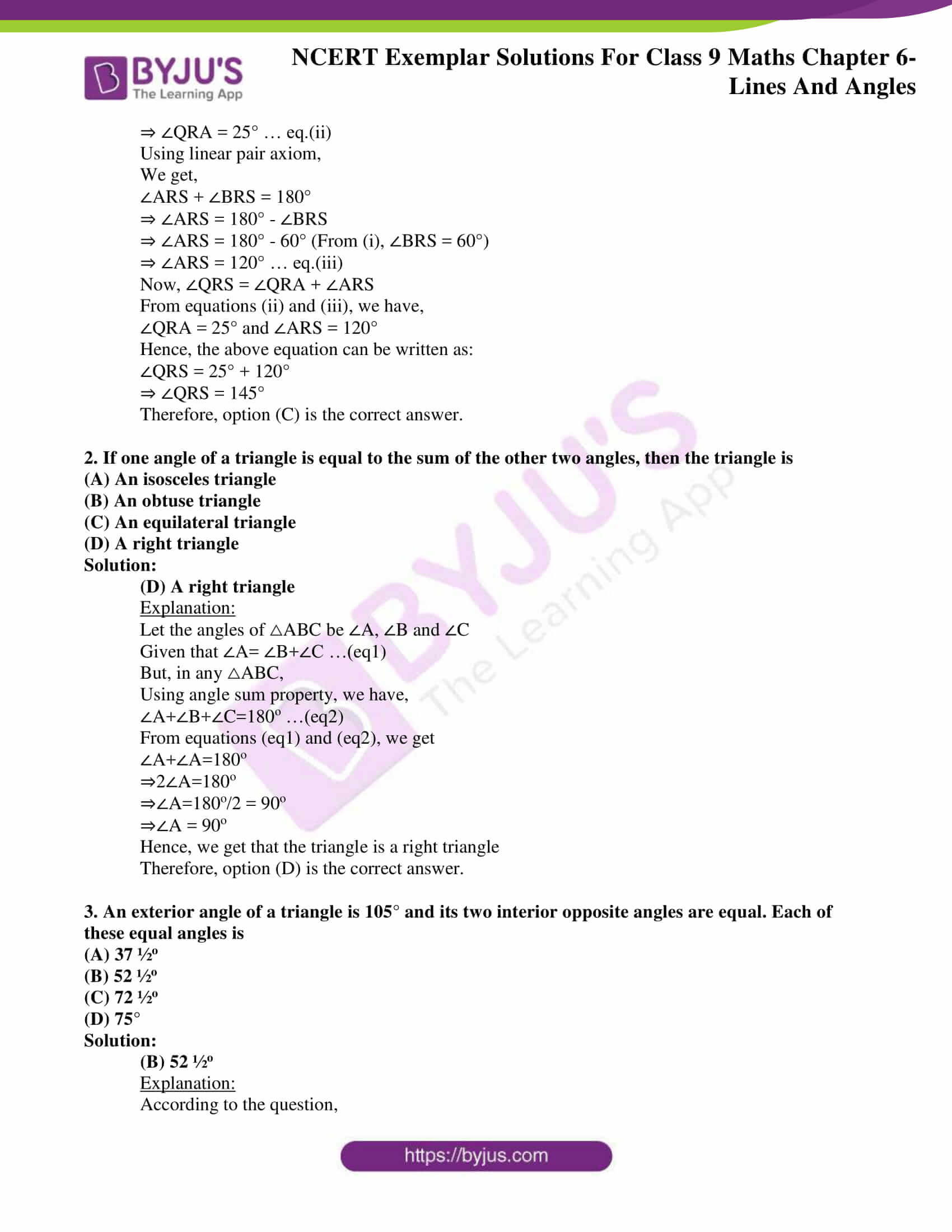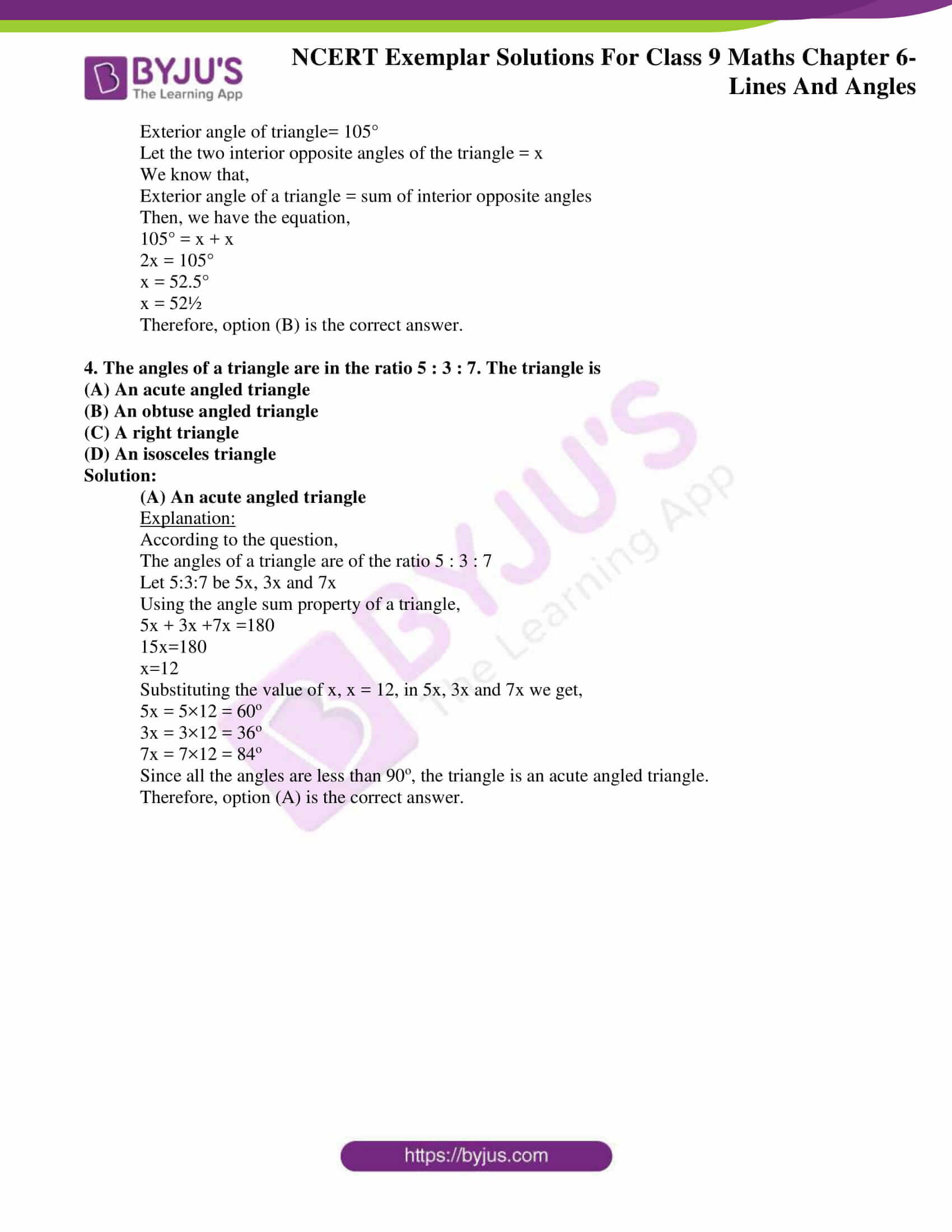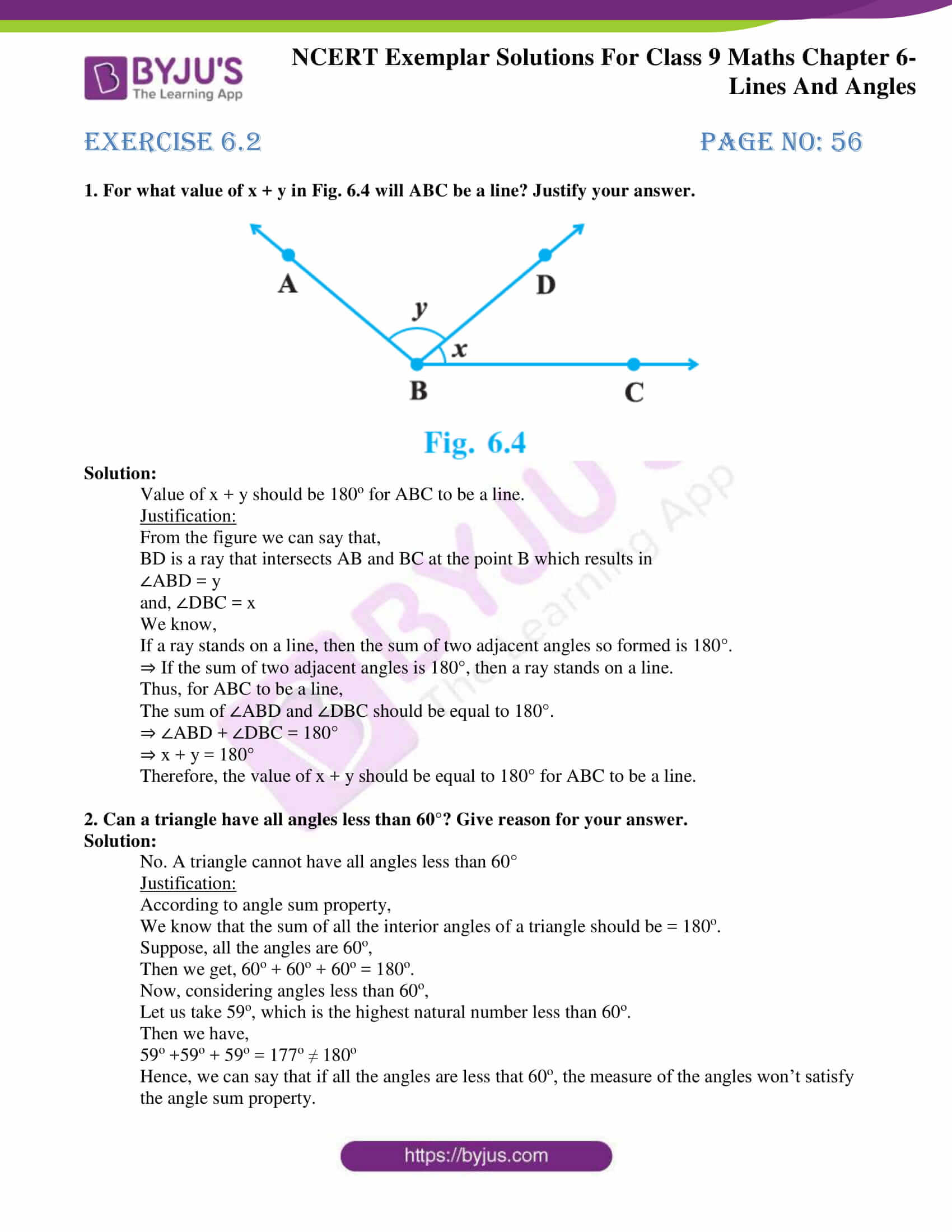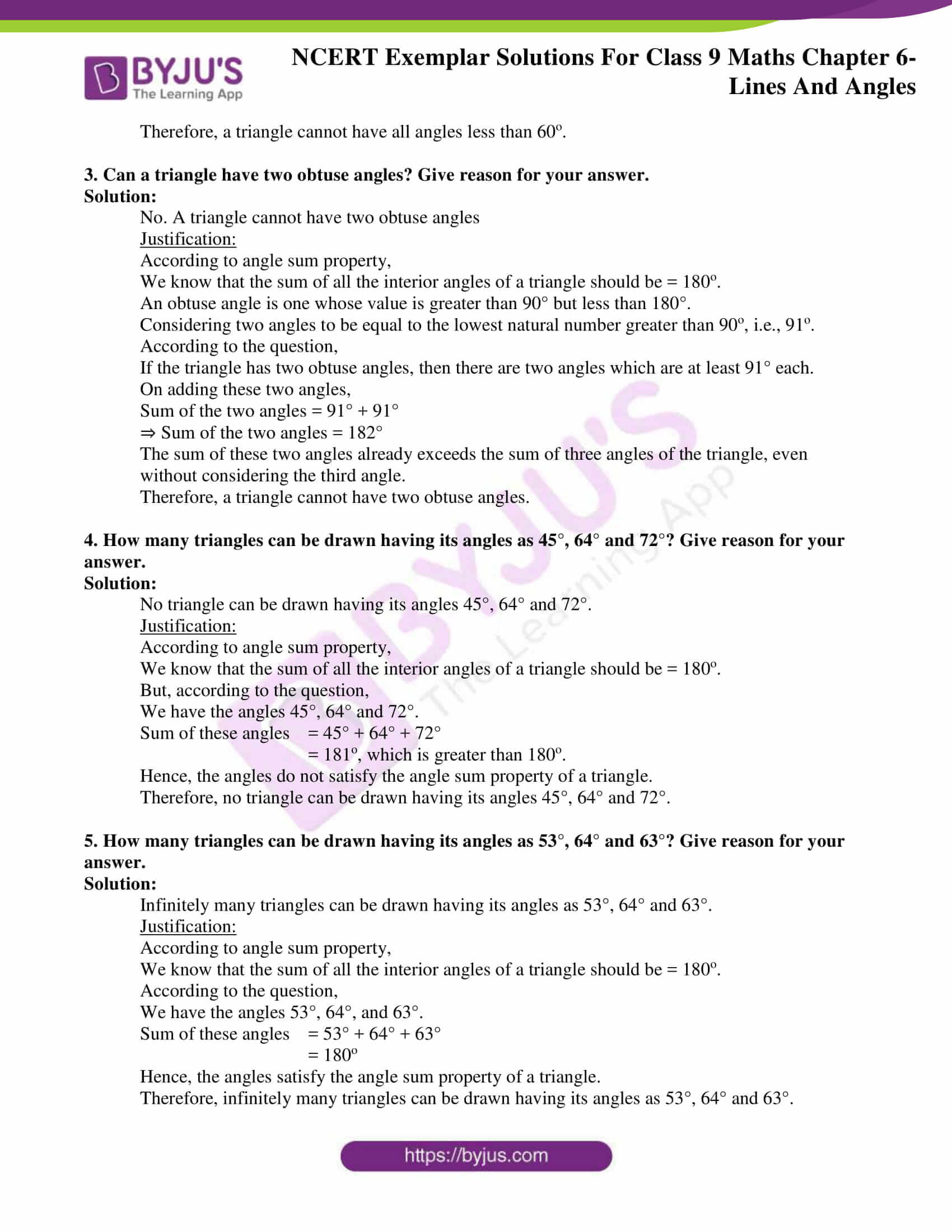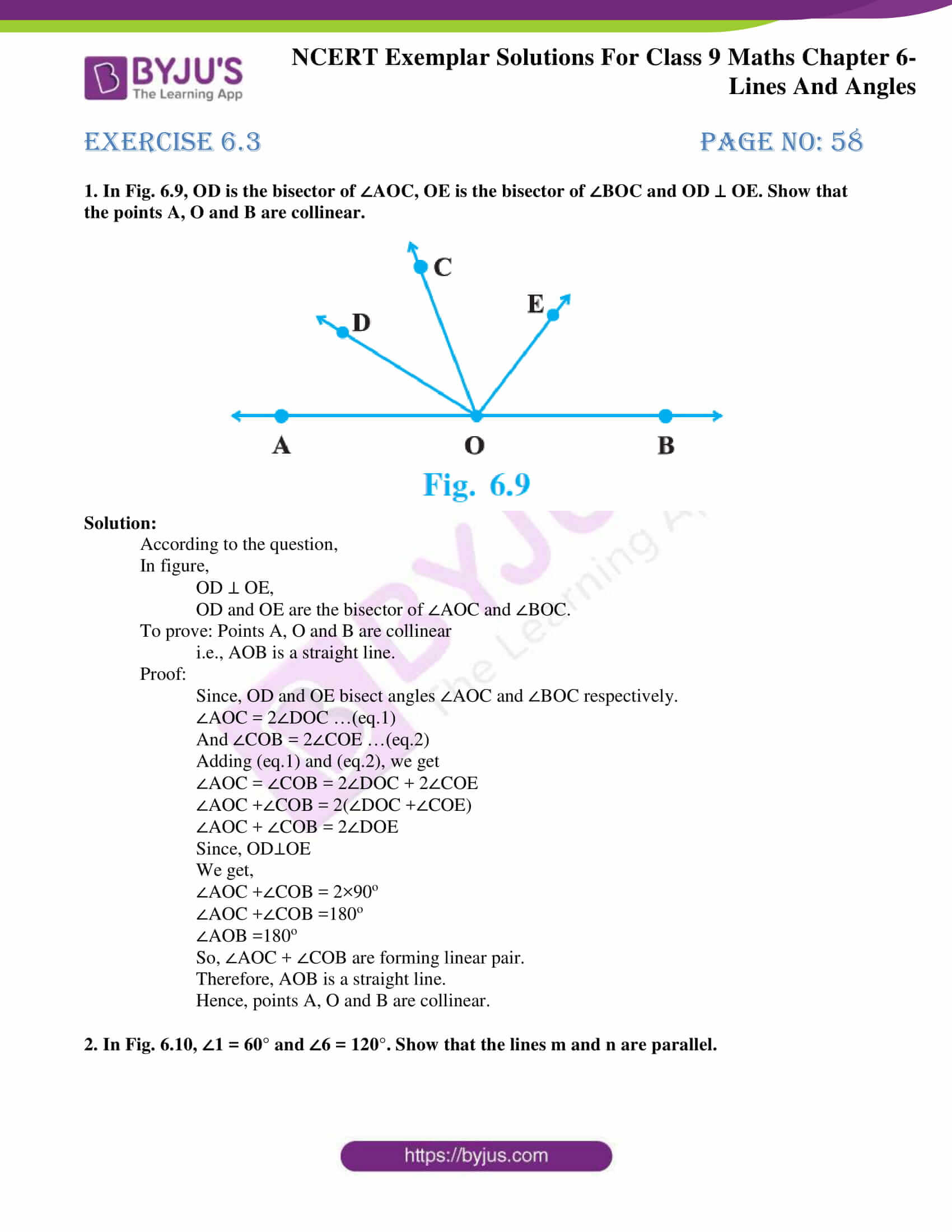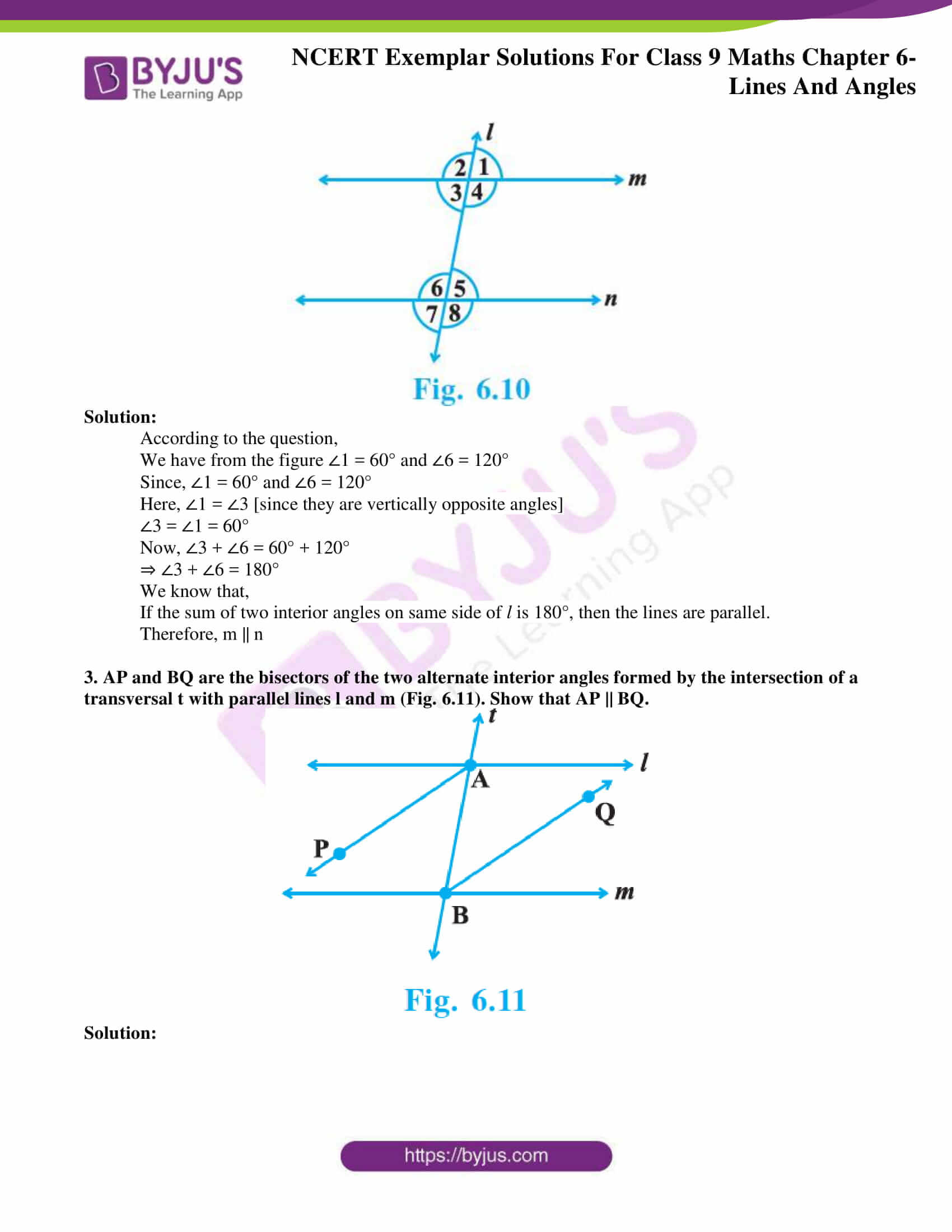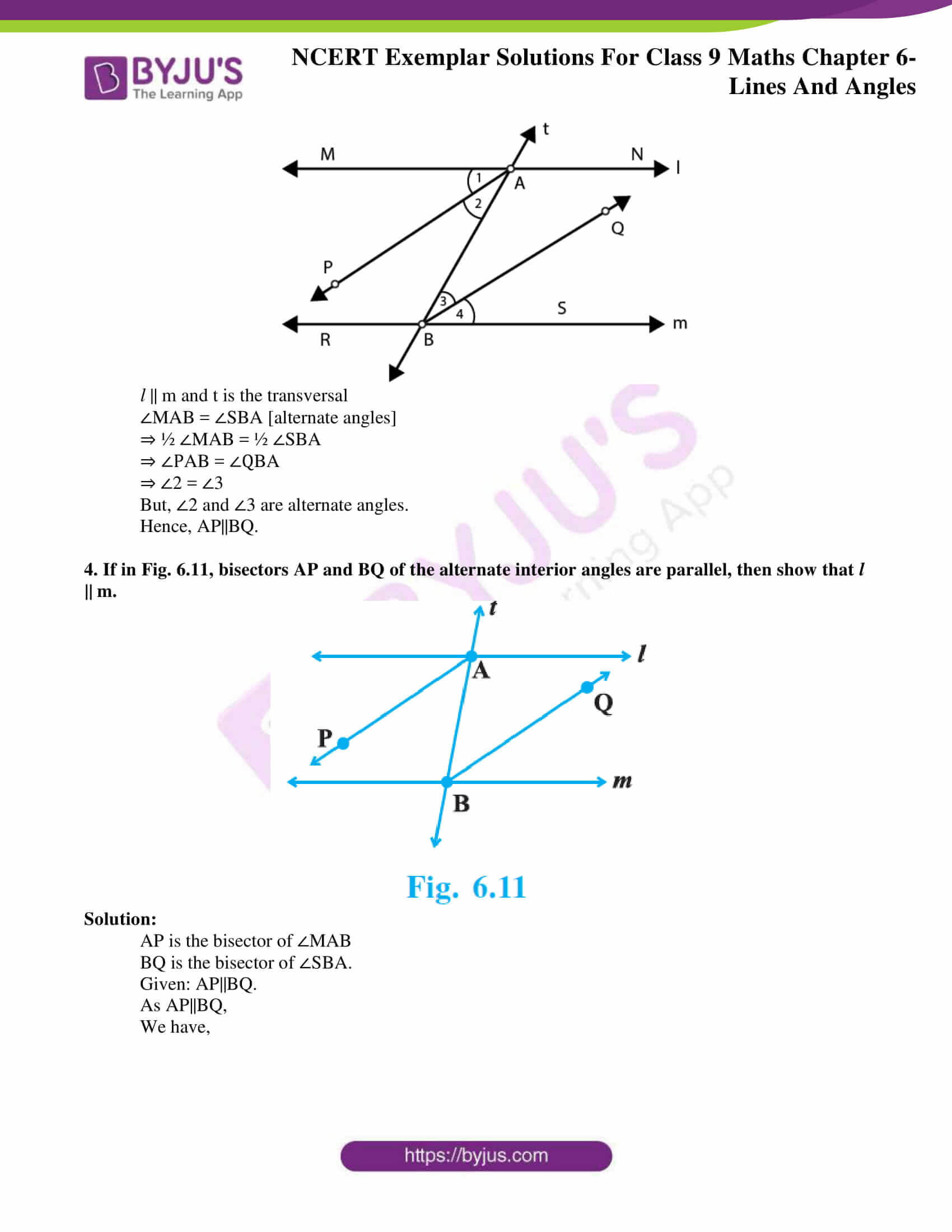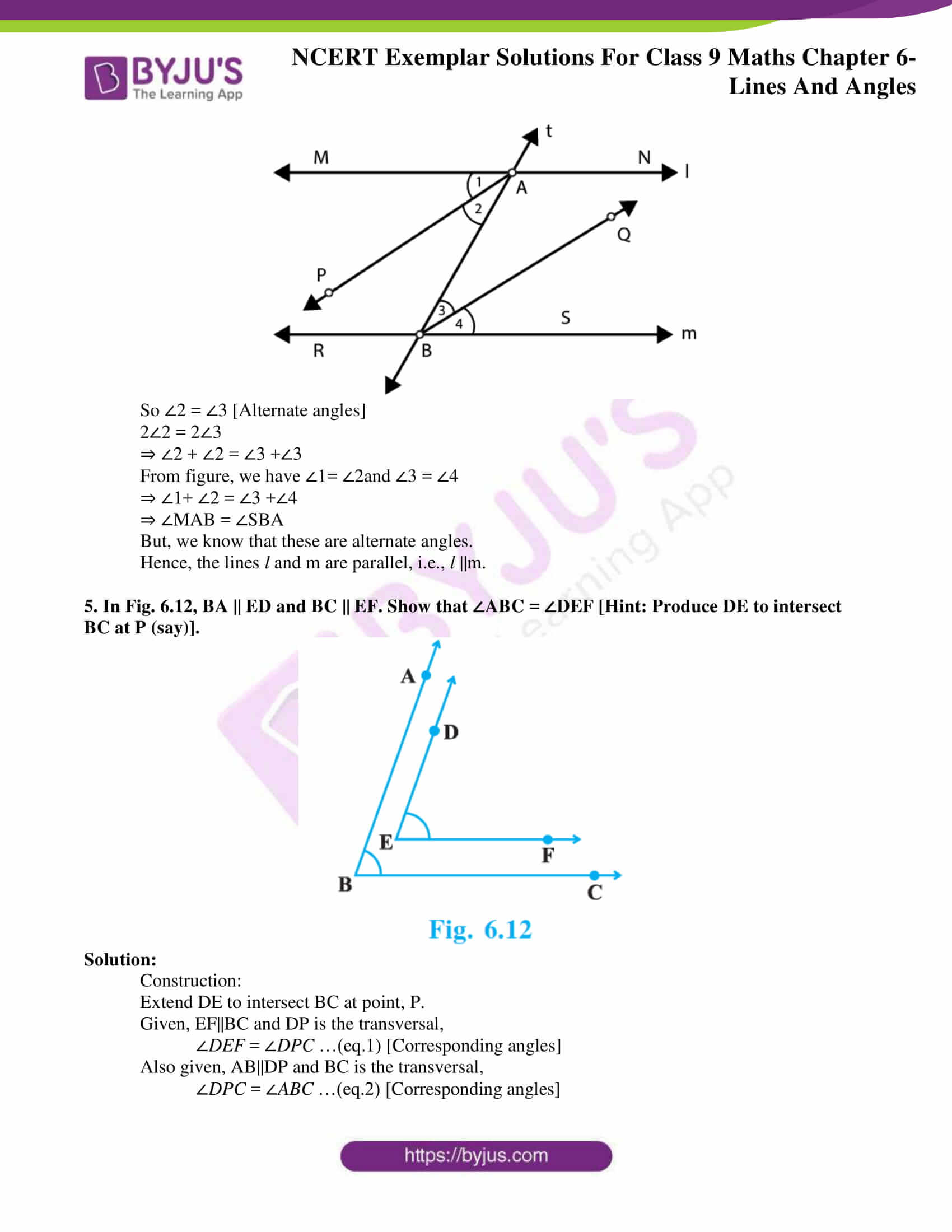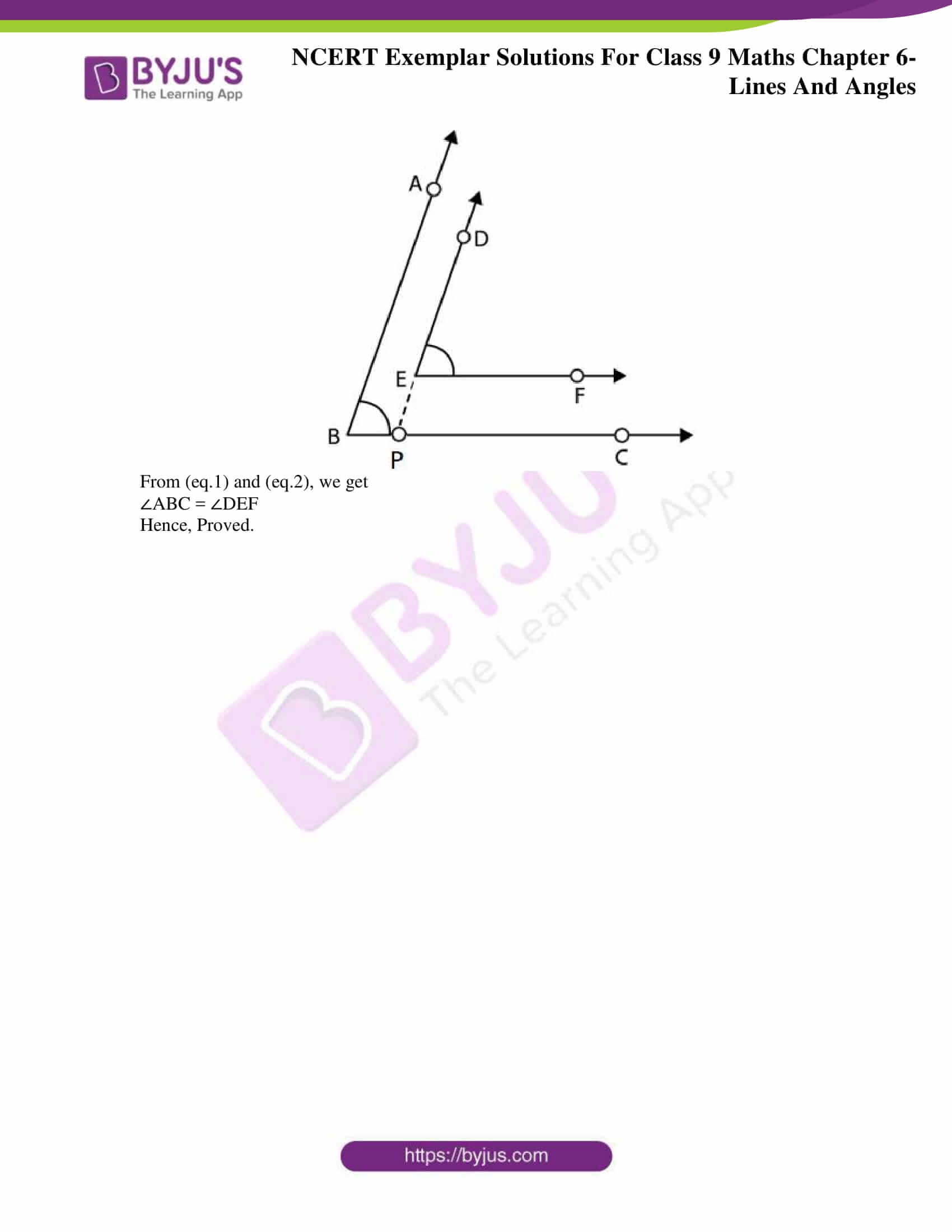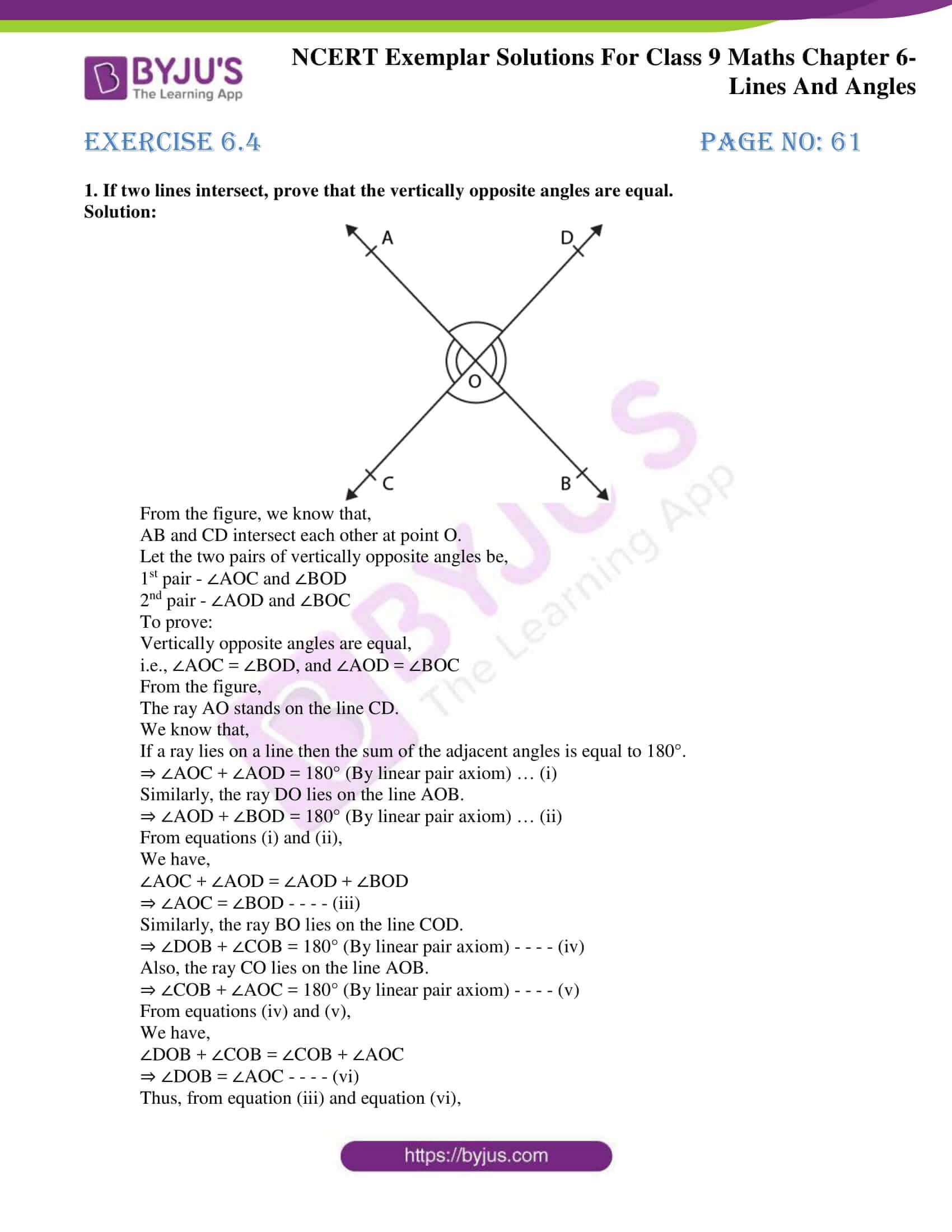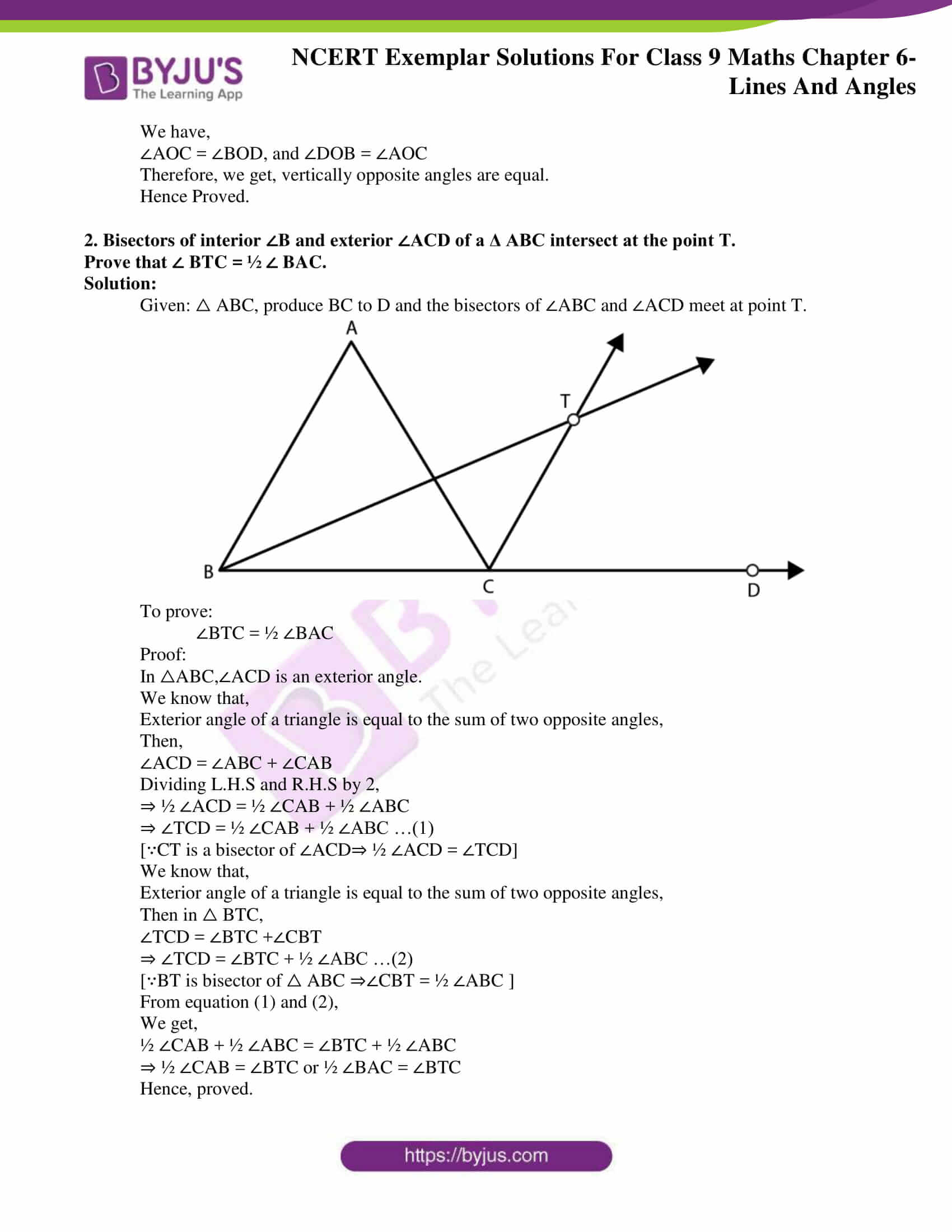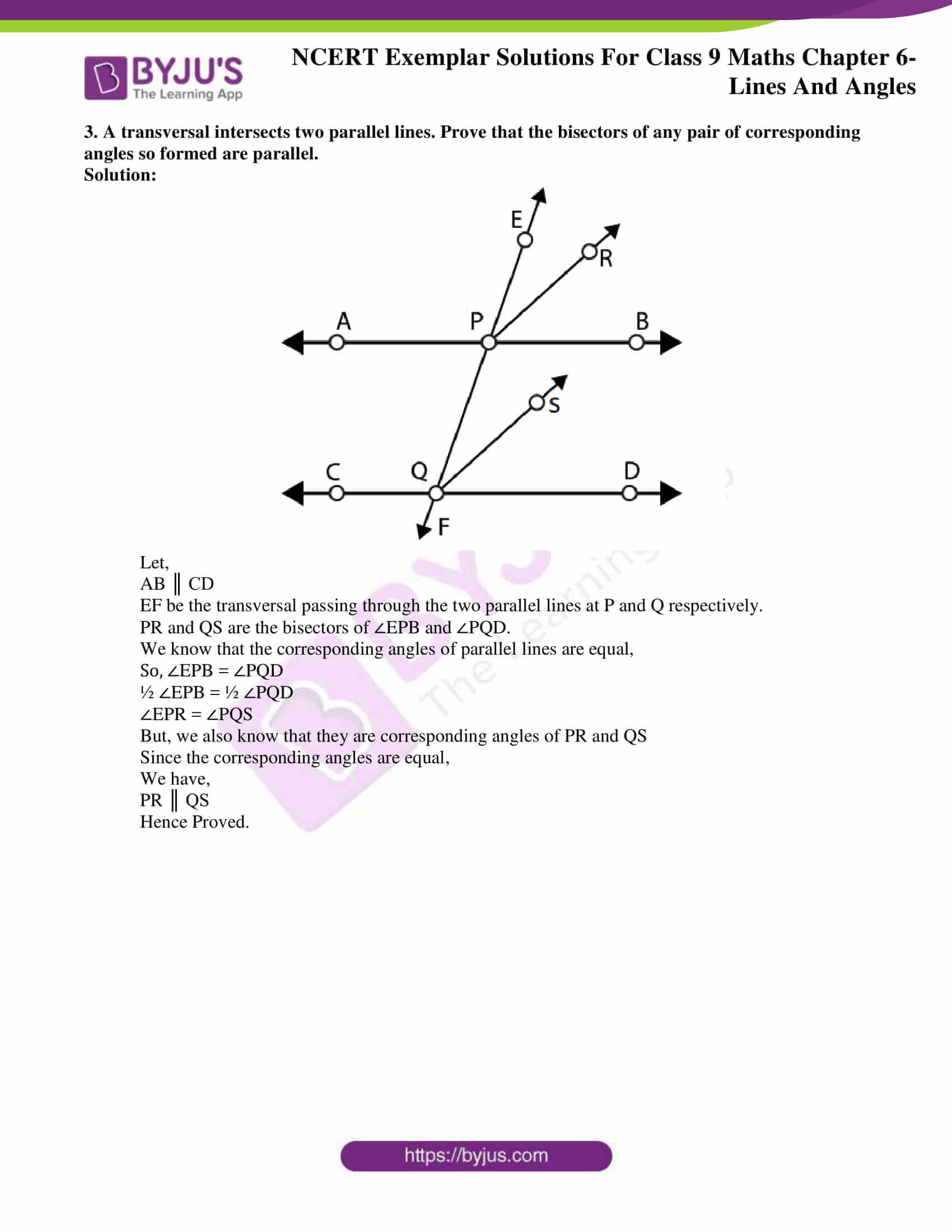### Access answers to NCERT Exemplar Solutions for Class 9 Maths Chapter 6 Lines and Angles

Exercise 6.1 Page No: 55

Write the correct answer in each of the following:

1. In Fig. 6.1, if AB || CD || EF, PQ || RS, ∠RQD = 25° and ∠CQP = 60°, then ∠QRS is equal to

(A) 85°

(B) 135°

(C) 145°

(D) 110°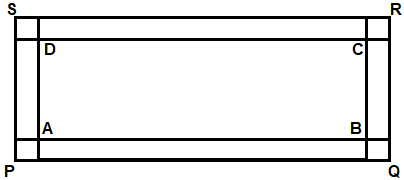Solution:

(C) 145°

Explanation:

According to the given figure, we have

AB || CD || EF

PQ || RS

∠RQD = 25°

∠CQP = 60°

PQ || RS.

We know that,

If a transversal intersects two parallel lines, then each pair of alternate exterior angles is equal.

Now, since, PQ || RS

⇒ ∠PQC = ∠BRS

We have ∠PQC = 60°

⇒ ∠BRS = 60° … eq.(i)

We also know that,

If a transversal intersects two parallel lines, then each pair of alternate interior angles is equal.

Now again, since, AB || CD

⇒ ∠DQR = ∠QRA

We have ∠DQR = 25°

⇒ ∠QRA = 25° … eq.(ii)

Using linear pair axiom,

We get,

∠ARS + ∠BRS = 180°

⇒ ∠ARS = 180° – ∠BRS

⇒ ∠ARS = 180° – 60° (From (i), ∠BRS = 60°)

⇒ ∠ARS = 120° … eq.(iii)

Now, ∠QRS = ∠QRA + ∠ARS

From equations (ii) and (iii), we have,

∠QRA = 25° and ∠ARS = 120°

Hence, the above equation can be written as:

∠QRS = 25° + 120°

⇒ ∠QRS = 145°

Therefore, option (C) is the correct answer.

2. If one angle of a triangle is equal to the sum of the other two angles, then the triangle is

(A) An isosceles triangle

(B) An obtuse triangle

(C) An equilateral triangle

(D) A right triangle

Solution:

(D) A right triangle

Explanation:

Let the angles of △ABC be ∠A, ∠B and ∠C

Given that ∠A= ∠B+∠C …(eq1)

But, in any △ABC,

Using angle sum property, we have,

∠A+∠B+∠C=180o …(eq2)

From equations (eq1) and (eq2), we get

∠A+∠A=180o

⇒2∠A=180o

⇒∠A=180o/2 = 90o

⇒∠A = 90o

Hence, we get that the triangle is a right triangle

Therefore, option (D) is the correct answer.

3. An exterior angle of a triangle is 105° and its two interior opposite angles are equal. Each of these equal angles is

(A) 37 ½o

(B) 52 ½o

(C) 72 ½o

(D) 75°

Solution:

(B) 52 ½o

Explanation:

According to the question,

Exterior angle of triangle= 105°

Let the two interior opposite angles of the triangle = x

We know that,

Exterior angle of a triangle = sum of interior opposite angles

Then, we have the equation,

105° = x + x

2x = 105°

x = 52.5°

x = 52½

Therefore, option (B) is the correct answer.

4. The angles of a triangle are in the ratio 5 : 3 : 7. The triangle is

(A) An acute angled triangle

(B) An obtuse angled triangle

(C) A right triangle

(D) An isosceles triangle

Solution:

(A) An acute angled triangle

Explanation:

According to the question,

The angles of a triangle are of the ratio 5 : 3 : 7

Let 5:3:7 be 5x, 3x and 7x

Using the angle sum property of a triangle,

5x + 3x +7x =180

15x=180

x=12

Substituting the value of x, x = 12, in 5x, 3x and 7x we get,

5x = 5×12 = 60o

3x = 3×12 = 36o

7x = 7×12 = 84o

Since all the angles are less than 90o, the triangle is an acute angled triangle.

Therefore, option (A) is the correct answer.

Exercise 6.2 Page No: 56

1. For what value of x + y in Fig. 6.4 will ABC be a line? Justify your answer.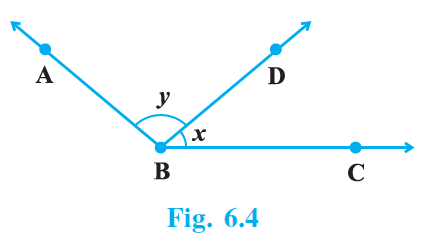Solution:

Value of x + y should be 180o for ABC to be a line.

Justification:

From the figure we can say that,

BD is a ray that intersects AB and BC at the point B which results in

∠ABD = y

and, ∠DBC = x

We know,

If a ray stands on a line, then the sum of two adjacent angles so formed is 180°.

⇒ If the sum of two adjacent angles is 180°, then a ray stands on a line.

Thus, for ABC to be a line,

The sum of ∠ABD and ∠DBC should be equal to 180°.

⇒ ∠ABD + ∠DBC = 180°

⇒ x + y = 180°

Therefore, the value of x + y should be equal to 180° for ABC to be a line.

2. Can a triangle have all angles less than 60°? Give reason for your answer.

Solution:

No. A triangle cannot have all angles less than 60°

Justification:

According to angle sum property,

We know that the sum of all the interior angles of a triangle should be = 180o.

Suppose, all the angles are 60o,

Then we get, 60o + 60o + 60o = 180o.

Now, considering angles less than 60o,

Let us take 59o, which is the highest natural number less than 60o.

Then we have,

59o +59o + 59o = 177o ≠ 180o

Hence, we can say that if all the angles are less that 60o, the measure of the angles won’t satisfy the angle sum property.

Therefore, a triangle cannot have all angles less than 60o.

3. Can a triangle have two obtuse angles? Give reason for your answer.

Solution:

No. A triangle cannot have two obtuse angles

Justification:

According to angle sum property,

We know that the sum of all the interior angles of a triangle should be = 180o.

An obtuse angle is one whose value is greater than 90° but less than 180°.

Considering two angles to be equal to the lowest natural number greater than 90o, i.e., 91o.

According to the question,

If the triangle has two obtuse angles, then there are two angles which are at least 91° each.

Sum of the two angles = 91° + 91°

⇒ Sum of the two angles = 182°

The sum of these two angles already exceeds the sum of three angles of the triangle, even without considering the third angle.

Therefore, a triangle cannot have two obtuse angles.

4. How many triangles can be drawn having its angles as 45°, 64° and 72°? Give reason for your answer.

Solution:

No triangle can be drawn having its angles 45°, 64° and 72°.

Justification:

According to angle sum property,

We know that the sum of all the interior angles of a triangle should be = 180o.

But, according to the question,

We have the angles 45°, 64° and 72°.

Sum of these angles = 45° + 64° + 72°

= 181o, which is greater than 180o.

Hence, the angles do not satisfy the angle sum property of a triangle.

Therefore, no triangle can be drawn having its angles 45°, 64° and 72°.

5. How many triangles can be drawn having its angles as 53°, 64° and 63°? Give reason for your answer.

Solution:

Infinitely many triangles can be drawn having its angles as 53°, 64° and 63°.

Justification:

According to angle sum property,

We know that the sum of all the interior angles of a triangle should be = 180o.

According to the question,

We have the angles 53°, 64°, and 63°.

Sum of these angles = 53° + 64° + 63°

= 180o

Hence, the angles satisfy the angle sum property of a triangle.

Therefore, infinitely many triangles can be drawn having its angles as 53°, 64° and 63°.

Exercise 6.3 Page No: 58

1. In Fig. 6.9, OD is the bisector of ∠AOC, OE is the bisector of ∠BOC and OD ⊥ OE. Show that the points A, O and B are collinear.Solution:

According to the question,

In figure,

OD ⊥ OE,

OD and OE are the bisector of ∠AOC and ∠BOC.

To prove: Points A, O and B are collinear

i.e., AOB is a straight line.

Proof:

Since, OD and OE bisect angles ∠AOC and ∠BOC respectively.

∠AOC = 2∠DOC …(eq.1)

And ∠COB = 2∠COE …(eq.2)

Adding (eq.1) and (eq.2), we get

∠AOC = ∠COB = 2∠DOC + 2∠COE

∠AOC +∠COB = 2(∠DOC +∠COE)

∠AOC + ∠COB = 2∠DOE

Since, OD⊥OE

We get,

∠AOC +∠COB = 2×90o

∠AOC +∠COB =180o

∠AOB =180o

So, ∠AOC + ∠COB are forming linear pair.

Therefore, AOB is a straight line.

Hence, points A, O and B are collinear.

2. In Fig. 6.10, ∠1 = 60° and ∠6 = 120°. Show that the lines m and n are parallel.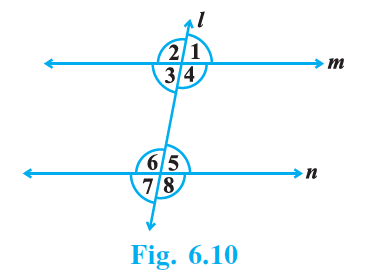Solution:

According to the question,

We have from the figure ∠1 = 60° and ∠6 = 120°

Since, ∠1 = 60° and ∠6 = 120°

Here, ∠1 = ∠3 [since they are vertically opposite angles]

∠3 = ∠1 = 60°

Now, ∠3 + ∠6 = 60° + 120°

⇒ ∠3 + ∠6 = 180°

We know that,

If the sum of two interior angles on same side of l is 180°, then the lines are parallel.

Therefore, m || n

3. AP and BQ are the bisectors of the two alternate interior angles formed by the intersection of a transversal t with parallel lines l and m (Fig. 6.11). Show that AP || BQ.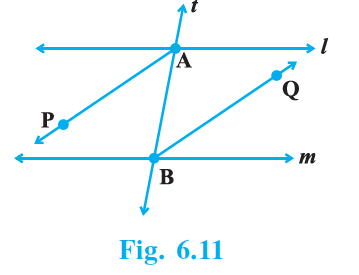Solution: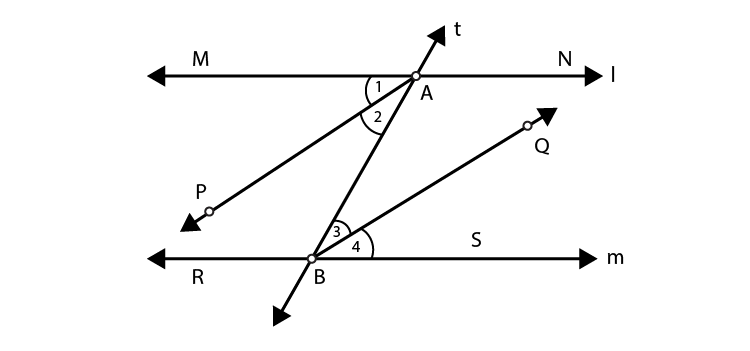l || m and t is the transversal

∠MAB = ∠SBA [alternate angles]

⇒ ½ ∠MAB = ½ ∠SBA

⇒ ∠PAB = ∠QBA

⇒ ∠2 = ∠3

But, ∠2 and ∠3 are alternate angles.

Hence, AP||BQ.

4. If in Fig. 6.11, bisectors AP and BQ of the alternate interior angles are parallel, then show that l || m.Solution:

AP is the bisector of ∠MAB

BQ is the bisector of ∠SBA.

Given: AP||BQ.

As AP||BQ,

We have,So ∠2 = ∠3 [Alternate angles]

2∠2 = 2∠3

⇒ ∠2 + ∠2 = ∠3 +∠3

From figure, we have ∠1= ∠2and ∠3 = ∠4

⇒ ∠1+ ∠2 = ∠3 +∠4

⇒ ∠MAB = ∠SBA

But, we know that these are alternate angles.

Hence, the lines l and m are parallel, i.e., l ||m.

5. In Fig. 6.12, BA || ED and BC || EF. Show that ∠ABC = ∠DEF [Hint: Produce DE to intersect BC at P (say)].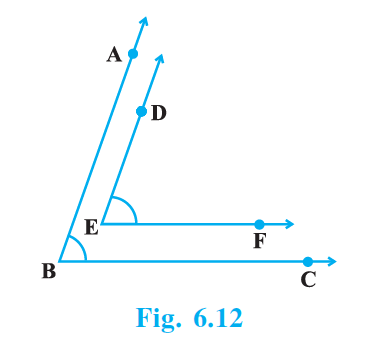Solution:

Construction:

Extend DE to intersect BC at point, P.

Given, EF||BC and DP is the transversal,

DEF = ∠DPC …(eq.1) [Corresponding angles]

Also given, AB||DP and BC is the transversal,

DPC = ∠ABC …(eq.2) [Corresponding angles]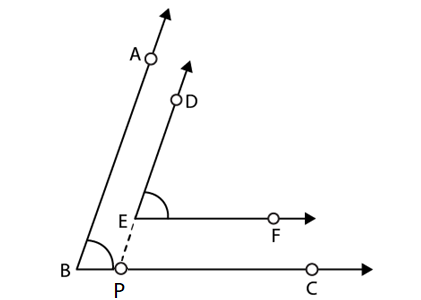From (eq.1) and (eq.2), we get

∠ABC = ∠DEF

Hence, Proved.

Exercise 6.4 Page No: 61

1. If two lines intersect, prove that the vertically opposite angles are equal.

Solution: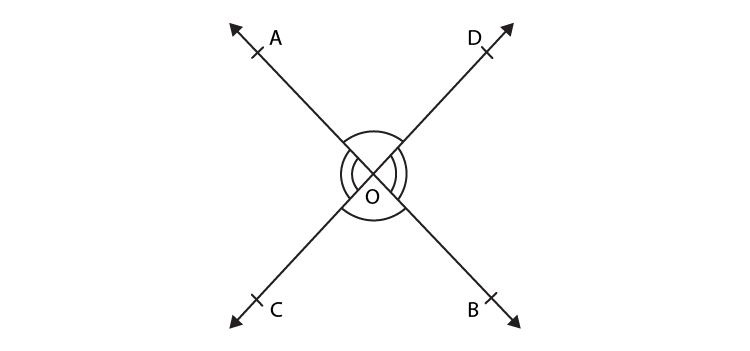From the figure, we know that,

AB and CD intersect each other at point O.

Let the two pairs of vertically opposite angles be,

1st pair – ∠AOC and ∠BOD

2nd pair – ∠AOD and ∠BOC

To prove:

Vertically opposite angles are equal,

i.e., ∠AOC = ∠BOD, and ∠AOD = ∠BOC

From the figure,

The ray AO stands on the line CD.

We know that,

If a ray lies on a line then the sum of the adjacent angles is equal to 180°.

⇒ ∠AOC + ∠AOD = 180° (By linear pair axiom) … (i)

Similarly, the ray DO lies on the line AOB.

⇒ ∠AOD + ∠BOD = 180° (By linear pair axiom) … (ii)

From equations (i) and (ii),

We have,

∠AOC + ∠AOD = ∠AOD + ∠BOD

⇒ ∠AOC = ∠BOD – – – – (iii)

Similarly, the ray BO lies on the line COD.

⇒ ∠DOB + ∠COB = 180° (By linear pair axiom) – – – – (iv)

Also, the ray CO lies on the line AOB.

⇒ ∠COB + ∠AOC = 180° (By linear pair axiom) – – – – (v)

From equations (iv) and (v),

We have,

∠DOB + ∠COB = ∠COB + ∠AOC

⇒ ∠DOB = ∠AOC – – – – (vi)

Thus, from equation (iii) and equation (vi),

We have,

∠AOC = ∠BOD, and ∠DOB = ∠AOC

Therefore, we get, vertically opposite angles are equal.

Hence Proved.

2. Bisectors of interior ∠B and exterior ∠ACD of a Δ ABC intersect at the point T.

Prove that ∠ BTC = ½ ∠ BAC.

Solution:

Given: △ ABC, produce BC to D and the bisectors of ∠ABC and ∠ACD meet at point T.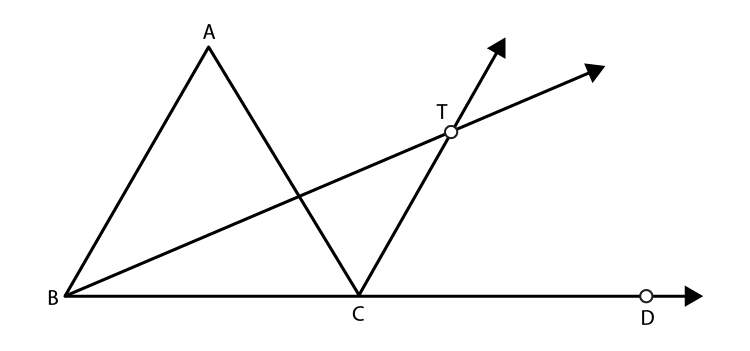To prove:

∠BTC = ½ ∠BAC

Proof:

In △ABC,∠ACD is an exterior angle.

We know that,

Exterior angle of a triangle is equal to the sum of two opposite angles,

Then,

∠ACD = ∠ABC + ∠CAB

Dividing L.H.S and R.H.S by 2,

⇒ ½ ∠ACD = ½ ∠CAB + ½ ∠ABC

⇒ ∠TCD = ½ ∠CAB + ½ ∠ABC …(1)

[∵CT is a bisector of ∠ACD⇒ ½ ∠ACD = ∠TCD]

We know that,

Exterior angle of a triangle is equal to the sum of two opposite angles,

Then in △ BTC,

∠TCD = ∠BTC +∠CBT

⇒ ∠TCD = ∠BTC + ½ ∠ABC …(2)

[∵BT is bisector of △ ABC ⇒∠CBT = ½ ∠ABC ]

From equation (1) and (2),

We get,

½ ∠CAB + ½ ∠ABC = ∠BTC + ½ ∠ABC

⇒ ½ ∠CAB = ∠BTC or ½ ∠BAC = ∠BTC

Hence, proved.

3. A transversal intersects two parallel lines. Prove that the bisectors of any pair of corresponding angles so formed are parallel.

Solution: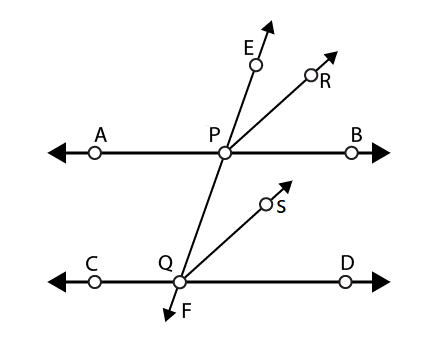Let,

AB ║ CD

EF be the transversal passing through the two parallel lines at P and Q respectively.

PR and QS are the bisectors of ∠EPB and ∠PQD.

We know that the corresponding angles of parallel lines are equal,

So, ∠EPB = ∠PQD

½ ∠EPB = ½ ∠PQD

∠EPR = ∠PQS

But, we also know that they are corresponding angles of PR and QS

Since the corresponding angles are equal,

We have,

PR ║ QS

Hence Proved.

Students of Class 9 can also use these exemplar solutions as a reference tool while practising the NCERT book exercise questions, which can also be downloaded in PDF form. Exemplar books, NCERT Solutions, notes and questions papers are also provided here, in BYJU’S as study materials for students to learn and practice for their final exams.

Sample papers and previous year question papers will help to know the question pattern and marks contained by Chapter 6 in Maths exam for Class 9. Also, solve important questions with NCERT Exemplar for chapter lines and angles. To download pdf click on the link below. Download BYJU’S-The learning app to get personalized videos teaching various concepts of Maths such as lines and angles and of related topics with the help of pictures and video contents.

## Frequently Asked Questions on NCERT Exemplar Solutions for Class 9 Maths Chapter 6

### What is the condition of collinearity of three points according to NCERT Exemplar Solutions for Class 9 Maths Chapter 6.

In general, lines can be parallel, perpendicular, intersection, etc. In all these cases, the slopes of lines are related to each other in some way. As we know, the slopes of two parallel lines are equal. If two lines have the same slope pass through a common point, then two lines will coincide. In other words, if A, B, and C are three points in the XY-plane, they will lie on a line, i.e., three points are collinear if and only if the slope of AB is equal to the slope of BC.

### Mention the important topics covered in the NCERT Exemplar Solutions for Class 9 Maths Chapter 6.

The important topics covered in the NCERT Exemplar Solutions for Class 9 Maths Chapter 6 are –
1. Basic terms like line-segment, collinear points, non-collinear points, right angle, straight angle, acute angle, reflex angle, complementary angles, etc.
2. Lines which are intersecting and non-intersecting
3. Linear pair of angles such as adjacent angles, vertically opposite angles, etc.
4. Transversal intersecting Parallel lines forming angles such as exterior angles, consecutive interior angles.
5. Lines parallel to the same line
6. Angle Sum Property of a Triangle

### What is meant by lines parallel to the same line covered in NCERT Exemplar Solutions for Class 9 Maths Chapter 6.

Lines that are parallel to the same line are parallel to each other. It means that if two lines are parallel to the same line, then they will be parallel to each other.